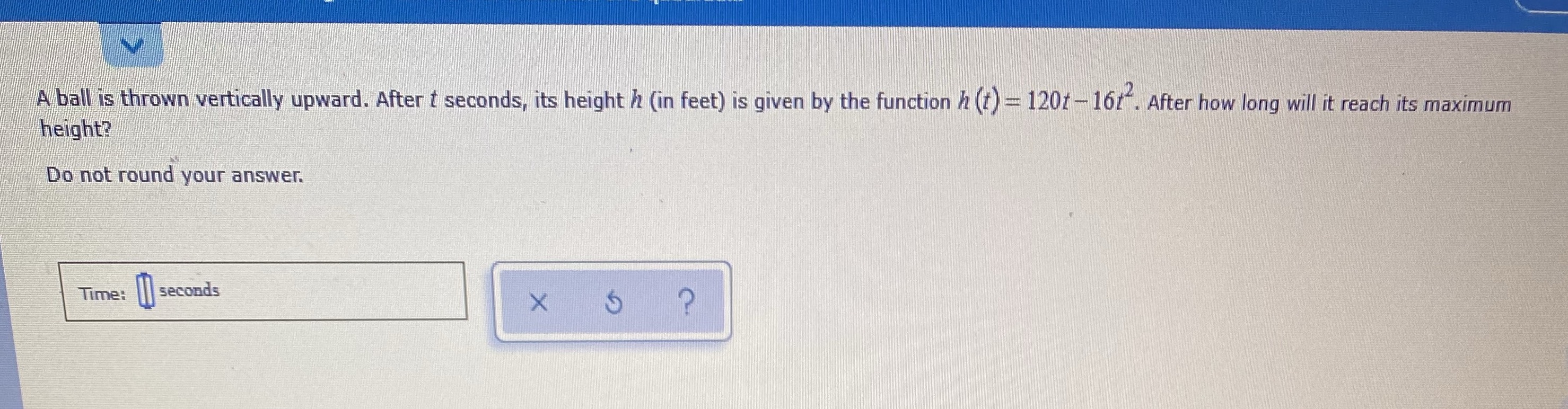### ¿Todavía tienes preguntas de matemáticas?

Pregunte a nuestros tutores expertos
Algebra
PreguntaA ball is thrown vertically upward. After $$t$$ seconds, its height $$h$$ (in feet) is given by the function $$h ( t ) = 120 t - 16 t ^ { 2 }$$ . After how long will it reach its maximum height? Do not round your answer.